# 8.6: Nonconservative Forces

•• OpenStax
• OpenStax
$$\newcommand{\vecs}{\overset { \rightharpoonup} {\mathbf{#1}} }$$ $$\newcommand{\vecd}{\overset{-\!-\!\rightharpoonup}{\vphantom{a}\smash {#1}}}$$$$\newcommand{\id}{\mathrm{id}}$$ $$\newcommand{\Span}{\mathrm{span}}$$ $$\newcommand{\kernel}{\mathrm{null}\,}$$ $$\newcommand{\range}{\mathrm{range}\,}$$ $$\newcommand{\RealPart}{\mathrm{Re}}$$ $$\newcommand{\ImaginaryPart}{\mathrm{Im}}$$ $$\newcommand{\Argument}{\mathrm{Arg}}$$ $$\newcommand{\norm}{\| #1 \|}$$ $$\newcommand{\inner}{\langle #1, #2 \rangle}$$ $$\newcommand{\Span}{\mathrm{span}}$$ $$\newcommand{\id}{\mathrm{id}}$$ $$\newcommand{\Span}{\mathrm{span}}$$ $$\newcommand{\kernel}{\mathrm{null}\,}$$ $$\newcommand{\range}{\mathrm{range}\,}$$ $$\newcommand{\RealPart}{\mathrm{Re}}$$ $$\newcommand{\ImaginaryPart}{\mathrm{Im}}$$ $$\newcommand{\Argument}{\mathrm{Arg}}$$ $$\newcommand{\norm}{\| #1 \|}$$ $$\newcommand{\inner}{\langle #1, #2 \rangle}$$ $$\newcommand{\Span}{\mathrm{span}}$$$$\newcommand{\AA}{\unicode[.8,0]{x212B}}$$

Learning Objectives

By the end of this section, you will be able to:

• Define nonconservative forces and explain how they affect mechanical energy.
• Show how the principle of conservation of energy can be applied by treating the conservative forces in terms of their potential energies and any nonconservative forces in terms of the work they do.

## Nonconservative Forces and Friction

Forces are either conservative or nonconservative. A nonconservative force is one for which work depends on the path taken. Friction is a good example of a nonconservative force. As illustrated in Figure $$\PageIndex{1}$$, work done against friction depends on the length of the path between the starting and ending points. Because of this dependence on path, there is no potential energy associated with nonconservative forces. An important characteristic is that the work done by a nonconservative force adds or removes mechanical energy from a system. Friction, for example, creates thermal energy that dissipates, removing energy from the system. Furthermore, even if the thermal energy is retained or captured, it cannot be fully converted back to work, so it is lost or not recoverable in that sense as well.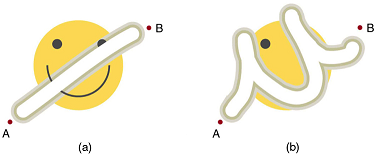Figure $$\PageIndex{1}$$: The amount of the happy face erased depends on the path taken by the eraser between points A and B, as does the work done against friction. Less work is done and less of the face is erased for the path in (a) than for the path in (b). The force here is friction, and most of the work goes into thermal energy that subsequently leaves the system (the happy face plus the eraser). The energy expended cannot be fully recovered.

## How Nonconservative Forces Affect Mechanical Energy

Mechanical energy may not be conserved when nonconservative forces act. For example, when a car is brought to a stop by friction on level ground, it loses kinetic energy, which is dissipated as thermal energy, reducing its mechanical energy. Figure compares the effects of conservative and nonconservative forces. We often choose to understand simpler systems such as that described in Figure $$\PageIndex{2a}$$ first before studying more complicated systems as in Figure $$\PageIndex{2b}$$.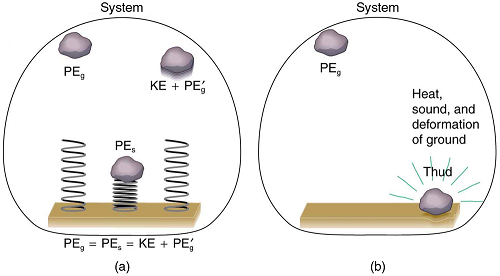Figure $$\PageIndex{2}$$: Comparison of the effects of conservative and nonconservative forces on the mechanical energy of a system. (a) A system with only conservative forces. When a rock is dropped onto a spring, its mechanical energy remains constant (neglecting air resistance) because the force in the spring is conservative. The spring can propel the rock back to its original height, where it once again has only potential energy due to gravity. (b) A system with nonconservative forces. When the same rock is dropped onto the ground, it is stopped by nonconservative forces that dissipate its mechanical energy as thermal energy, sound, and surface distortion. The rock has lost mechanical energy.

## How the Work-Energy Theorem Applies

Now let us consider what form the work-energy theorem takes when both conservative and nonconservative forces act. We will see that the work done by nonconservative forces equals the change in the mechanical energy of a system. As noted in Kinetic Energy and the Work-Energy Theorem, the work-energy theorem states that the net work on a system equals the change in its kinetic energy, or $$W_{net} = \Delta KE$$. The net work is the sum of the work by nonconservative forces plus the work by conservative forces. That is,

$W_{net} = W_{nc} + W_c,$

so that

$W_{nc} + W_c = \Delta KE,$

where $$W_{nc}$$ is the total work done by all nonconservative forces and $$W_c$$ is the total work done by all conservative forces.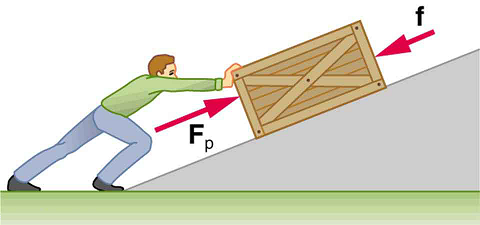Figure $$\PageIndex{3}$$: A person pushes a crate up a ramp, doing work on the crate. Friction and gravitational force (not shown) also do work on the crate; both forces oppose the person’s push. As the crate is pushed up the ramp, it gains mechanical energy, implying that the work done by the person is greater than the work done by friction.

Consider Figure $$\PageIndex{3}$$, in which a person pushes a crate up a ramp and is opposed by friction. As in the previous section, we note that work done by a conservative force comes from a loss of gravitational potential energy, so that $$W_c = -\Delta PE$$. Substituting this equation into the previous one and solving for $$W_{nc}$$ gives

$W_{nc} = \Delta KE + \Delta PE.\label{eq4}$

This equation means that the total mechanical energy $$(KE + PE)$$ changes by exactly the amount of work done by nonconservative forces. In Figure $$\PageIndex{3}$$, this is the work done by the person minus the work done by friction. So even if energy is not conserved for the system of interest (such as the crate), we know that an equal amount of work was done to cause the change in total mechanical energy. We rearrange Equation \ref{eq5} to obtain

$KE_i + PE_i + W_{nc} = KE_f + PE_f \label{eq5}$

This means that the amount of work done by nonconservative forces adds to the mechanical energy of a system. If $$W_{nc}$$ is positive, then mechanical energy is increased, such as when the person pushes the crate up the ramp in Figure. If $$W_{nc}$$ is negative, then mechanical energy is decreased, such as when the rock hits the ground in Figure $$\PageIndex{2b}$$. If $$W_{nc}$$ is negative, then mechanical energy is decreased, such as when the rock hits the ground in Figure $$\PageIndex{2b}$$. If $$W_{nc}$$ is zero, then mechanical energy is conserved, and nonconservative forces are balanced. For example, when you push a lawn mower at constant speed on level ground, your work done is removed by the work of friction, and the mower has a constant energy.

## Applying Energy Conservation with Nonconservative Forces

When no change in potential energy occurs, applying Equation \ref{eq5} amounts to applying the work-energy theorem by setting the change in kinetic energy to be equal to the net work done on the system, which in the most general case includes both conservative and nonconservative forces. But when seeking instead to find a change in total mechanical energy in situations that involve changes in both potential and kinetic energy, the previous equation $$KE_i + PE_i + W_{nc} = KE_f + PE_f$$ says that you can start by finding the change in mechanical energy that would have resulted from just the conservative forces, including the potential energy changes, and add to it the work done, with the proper sign, by any nonconservative forces involved.

Example $$\PageIndex{1}$$: Calculating Distance Traveled: How Far a Baseball Player Slides

Consider the situation shown in Figure $$\PageIndex{4}$$, where a baseball player slides to a stop on level ground. Using energy considerations, calculate the distance the 65.0-kg baseball player slides, given that his initial speed is 6.00 m/s and the force of friction against him is a constant 450 N.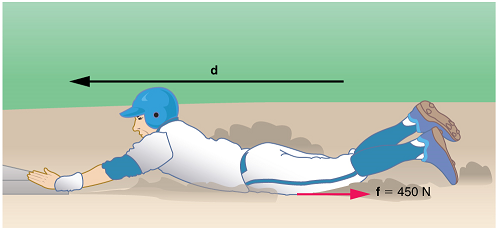Figure $$\PageIndex{4}$$: The baseball player slides to a stop in a distance d. In the process, friction removes the player’s kinetic energy by doing an amount of work $$fd$$ equal to the initial kinetic energy.

Strategy

Friction stops the player by converting his kinetic energy into other forms, including thermal energy. In terms of the work-energy theorem, the work done by friction, which is negative, is added to the initial kinetic energy to reduce it to zero. The work done by friction is negative, because f is in the opposite direction of the motion (that is, $$\theta = 180^o$$, and so $$cos \, \theta = -1$$). Thus, $$W_{nc} = -fd$$. The equation simplifies to

$\dfrac{1}{2} mv_i^2 - bfd = 0 \nonumber$

or

$fd = \dfrac{1}{2}mv_i^2. \nonumber$

This equation can now be solved for the distance $$d$$.

Solution

Solving the previous equation for $$d$$ and substituting known values yields

\begin{align*} d &= \dfrac{mv_i^2}{2f} \\[5pt] &= \dfrac{65.0 \, kg)(6.00 \, m/s)^2}{(2)(450 \, N)} \\[5pt] &= 2.60 \, m. \end{align*}

Discussion

The most important point of this example is that the amount of nonconservative work equals the change in mechanical energy. For example, you must work harder to stop a truck, with its large mechanical energy, than to stop a mosquito.

Example $$\PageIndex{2}$$: Calculating Distance Traveled: Sliding Up an Incline

Suppose that the player from Example $$\PageIndex{1}$$ is running up a hill having a $$5.00^o$$ incline upward with a surface similar to that in the baseball stadium. The player slides with the same initial speed, and the frictional force is still 450 N. Determine how far he slides.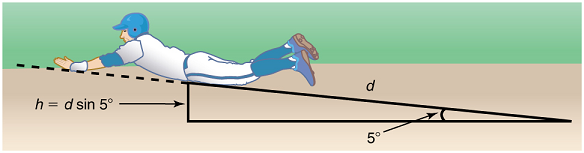Figure $$\PageIndex{5}$$: The same baseball player slides to a stop on a $$5.00^o$$ slope.

Strategy

In this case, the work done by the nonconservative friction force on the player reduces the mechanical energy he has from his kinetic energy at zero height, to the final mechanical energy he has by moving through distance $$d$$ to reach height $$h$$ along the hill, with $$h = d \, \sin \, 5.00^o$$. This is expressed by Equation \ref{eq5}

$KE_i + PE_i + W_{nc} = KE_f + PE_f . \nonumber$

Solution

The work done by friction is again $$W_{nc} = -fd$$; initially the potential energy is $$PE_i = mg \cdot 0 = 0$$ and the kinetic energy is $$KE_i = \frac{1}{2} \, mv^2$$; the final energy contributions are $$KE_f = 0$$ for the kinetic energy and $$PE_f = mgh = mgd \, sin \, \theta$$ for the potential energy.

Substituting these values gives

$\nonumber \dfrac{1}{2} mv_i^2 + 0 + (-fd) = 0 + mgd \, \sin \, \theta.$

Solve this for $$d$$ to obtain

\begin{align*} d &= \dfrac{\frac{1}{2} mv_i^2}{f + mg \, \sin \, \theta} \\[5pt] &= \dfrac{(0.5)(65.0 \, kg)(6.00 \, m/s)^2}{450 \, N + (65.0 \, kg)(9.80 \, m/s^2)sin \, (5.00^o)} \\[5pt] &= 2.31 \, m. \end{align*}

Discussion

As might have been expected, the player slides a shorter distance by sliding uphill. Note that the problem could also have been solved in terms of the forces directly and the work energy theorem, instead of using the potential energy. This method would have required combining the normal force and force of gravity vectors, which no longer cancel each other because they point in different directions, and friction, to find the net force. You could then use the net force and the net work to find the distance $$d$$ that reduces the kinetic energy to zero. By applying conservation of energy and using the potential energy instead, we need only consider the gravitational potential energy $$mgh$$, without combining and resolving force vectors. This simplifies the solution considerably.

Making Connections: Take-Home Investigation - Determining Friction from the Stopping Distance

This experiment involves the conversion of gravitational potential energy into thermal energy. Use the ruler, book, and marble from Take-Home Investigation—Converting Potential to Kinetic Energy. In addition, you will need a foam cup with a small hole in the side, as shown in Figure $$\PageIndex{6}$$. From the 10-cm position on the ruler, let the marble roll into the cup positioned at the bottom of the ruler. Measure the distance $$d$$ the cup moves before stopping. What forces caused it to stop? What happened to the kinetic energy of the marble at the bottom of the ruler? Next, place the marble at the 20-cm and the 30-cm positions and again measure the distance the cup moves after the marble enters it. Plot the distance the cup moves versus the initial marble position on the ruler. Is this relationship linear? With some simple assumptions, you can use these data to find the coefficient of kinetic friction $$\mu_k$$ of the cup on the table. The force of friction $$f$$ on the cup is $$\mu_k N$$, where the normal force $$N$$ is just the weight of the cup plus the marble. The normal force and force of gravity do no work because they are perpendicular to the displacement of the cup, which moves horizontally. The work done by friction is $$fd$$. You will need the mass of the marble as well to calculate its initial kinetic energy.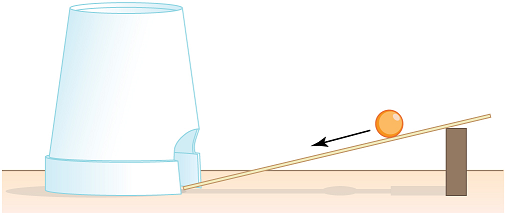Figure $$\PageIndex{6}$$: Rolling a marble down a ruler into a foam cup.

It is interesting to do the above experiment also with a steel marble (or ball bearing). Releasing it from the same positions on the ruler as you did with the glass marble, is the velocity of this steel marble the same as the velocity of the marble at the bottom of the ruler? Is the distance the cup moves proportional to the mass of the steel and glass marbles?

Phet Explorations: The Ramp

Explore forces, energy and work as you push household objects up and down a ramp. Lower and raise the ramp to see how the angle of inclination affects the parallel forces acting on the file cabinet. Graphs show forces, energy and work.Figure $$\PageIndex{7}$$: The Ramp

## Summary

• A nonconservative force is one for which work depends on the path.
• Friction is an example of a nonconservative force that changes mechanical energy into thermal energy.
• Work $$W_{nc}$$ done by a nonconservative force changes the mechanical energy of a system. In equation form, $$W_{nc} = \Delta KE + \Delta PE$$ or, equivalently, $$KE_i + PE_i + W_{nc} = KE_f + PE_f .$$
• When both conservative and nonconservative forces act, energy conservation can be applied and used to calculate motion in terms of the known potential energies of the conservative forces and the work done by nonconservative forces, instead of finding the net work from the net force, or having to directly apply Newton’s laws

## Glossary

nonconservative force
a force whose work depends on the path followed between the given initial and final configurations
friction
the force between surfaces that opposes one sliding on the other; friction changes mechanical energy into thermal energy

This page titled 8.6: Nonconservative Forces is shared under a CC BY license and was authored, remixed, and/or curated by OpenStax.